Advertisement Remove all ads

# Goyal Brothers Prakashan solutions for Class 9 A New Approach to ICSE Physics Part 1 chapter 2 - Motion in One Dimension [Latest edition]

#### ChaptersAdvertisement Remove all ads
Advertisement Remove all ads
Advertisement Remove all ads

## Chapter 2: Motion in One Dimension

Unit I Exercise 1ExerciseUnit I Practice Problems 1Unit I Practice Problems 2Unit II Exercise 2 (A) Objective QuestionsUnit II Exercise 2 (B) Subjective QuestionsUnit II Practice Problems 1Unit II Practice Problems 2Unit II Practice Problems 3Unit II Practice Problems 4Unit III Practice Problems 1Unit III Practice Problems 2Unit III Practice Problems 3Unit III Practice Problems 4Unit III Practice Problems 5Unit III Practice Problems 6Unit III Practice Problems 7Unit III Practice Problems 8
Advertisement Remove all ads
Unit I Exercise 1, Exercise [Page 61]

### Goyal Brothers Prakashan solutions for Class 9 A New Approach to ICSE Physics Part 1 Chapter 2 Motion in One DimensionUnit I Exercise 1, Exercise [Page 61]

Unit I Exercise 1 | Q 1.1

What do you understand by the term rest? Support your answer by giving two examples

Unit I Exercise 1 | Q 1.2

What do you understand by the term motion? Support your answer by giving two examples

Unit I Exercise 1 | Q 2

By giving an example, prove that rest and motion are relative terms.

Exercise | Q 3 | Page 61

Is mass a vector quantity?

Unit I Exercise 1 | Q 3.1

Define Scalar quantities.

Unit I Exercise 1 | Q 3.2

Define Vector quantities.

Unit I Exercise 1 | Q 3.3

Differentiate between the scalar and vector quantities, giving two examples of each.

Unit I Exercise 1 | Q 4

Pick out the scalar and vector quantities from the following list:

1. mass
2. density
3. displacement
4. distance
5. momentum
6. acceleration
7. temperature
8. time
Unit I Exercise 1 | Q 5.1

Define Speed.

Unit I Exercise 1 | Q 5.2

Define Velocity.

Unit I Exercise 1 | Q 5.3

Give two differences between speed and velocity.

Unit I Exercise 1 | Q 6.1

Define Distance.

Unit I Exercise 1 | Q 6.2

Define Displacement.

Unit I Exercise 1 | Q 6.3

Give two differences between displacement and distance.

Unit I Exercise 1 | Q 7.1

Define variable velocity and give one example.

Unit I Exercise 1 | Q 7.2

Define average velocity and give one example.

Unit I Exercise 1 | Q 7.3

Define uniform velocity and give one example.

Unit I Exercise 1 | Q 8.1

What do you understand by the term acceleration?

Unit I Exercise 1 | Q 8.2

When is the positive acceleration?

Unit I Exercise 1 | Q 8.2

When is the negative acceleration?

Unit I Exercise 1 | Q 9.1

Define the term acceleration due to gravity. State its average value.

Unit I Exercise 1 | Q 9.2

State its value in C.G.S. as well as in S.I. system.

Unit I Exercise 1 | Q 9.3

When is the acceleration due to gravity positive?

Unit I Exercise 1 | Q 9.3

When is the acceleration due to gravity negative?

Unit I Exercise 1 | Q 10

Give an example of a body which covers a certain distance, but its displacement is zero.

Unit I Exercise 1 | Q 11

Give an example of an accelerated body, moving with a uniform speed.

Unit I Exercise 1 | Q 12.1

What is the relation between distance and time when the body is moving with uniform velocity?

Unit I Exercise 1 | Q 12.2

What is the relation between distance and time when the body is moving with variable velocity?

Unit I Exercise 1 | Q 13.1

Differentiate between the scalar and vector quantities, giving two examples of each.

Unit I Exercise 1 | Q 13.2

State whether the following is scalar or vector quantity.

Speed

Unit I Exercise 1 | Q 13.2

State whether the following is scalar or vector quantity

Force

Unit I Exercise 1 | Q 13.2

State whether the following is scalar or vector quantity

Acceleration

Unit I Exercise 1 | Q 13.2

State whether the following is scalar or vector quantity

Energy

Unit I Exercise 1 | Q 14.1

Copy the following table and fill in the blank spaces.

 Quantity S.I. Unit Scalar or Vector Displacement ___________ ___________
Unit I Exercise 1 | Q 14.2

Copy the following table and fill in the blank spaces.

 Quantity S.I. Unit Scalar or Vector ___________ kg m−3 ___________
Unit I Exercise 1 | Q 15

Draw a diagram to show the motion of a body whose speed remains constant, but velocity changes continuously.

Unit I Practice Problems 1

### Goyal Brothers Prakashan solutions for Class 9 A New Approach to ICSE Physics Part 1 Chapter 2 Motion in One DimensionUnit I Practice Problems 1

Unit I Practice Problems 1 | Q 1

A car covers 90 km in 1 1/2 hours towards east. Calculate

1. displacement of car,
2. its velocity in (a) kmh−1 (b) ms−1.
Unit I Practice Problems 1 | Q 2

A racehorse runs straight towards the north and covers 540 m in one minute. Calculate

1. displacement of the horse,
2. its velocity in (a) ms−1 (b) kmh−1.
Unit I Practice Problems 2

### Goyal Brothers Prakashan solutions for Class 9 A New Approach to ICSE Physics Part 1 Chapter 2 Motion in One DimensionUnit I Practice Problems 2

Unit I Practice Problems 2 | Q 1

The change in velocity of a motorbike is 54 kmh−1 in one minute. Calculate its acceleration in (a) ms−2 (b) kmh−2.

Unit I Practice Problems 2 | Q 2

A speeding car changes its velocity from 108 kmh−1 to 36 kmh−1 in 4 s. Calculate its deceleration in

1. ms−2
2. kmh−2.
Unit II Exercise 2 (A) Objective Questions

### Goyal Brothers Prakashan solutions for Class 9 A New Approach to ICSE Physics Part 1 Chapter 2 Motion in One DimensionUnit II Exercise 2 (A) Objective Questions

Unit II Exercise 2 (A) Objective Questions | Q 1

Multiple choice Question. Select the correct option.

A graph is a straight line parallel to the time axis in a distance-time graph. From the graph, it implies:

• body is stationary

• body is moving with a uniform speed

• body is moving with a variable speed

• none of these

Unit II Exercise 2 (A) Objective Questions | Q 2

Multiple choice Question. Select the correct option.

The slope of displacement – time graph represents.

• uniform speed

• non-uniform speed

• uniform velocity

• uniform a acceleration

Unit II Exercise 2 (A) Objective Questions | Q 3

Multiple choice Question. Select the correct option.

A body dropped from the top of a tower reaches the ground in 4s. Height of the tower is

• 39.2 m

• 44.1 m

• 58.8 m

• 78.4 m

Unit II Exercise 2 (A) Objective Questions | Q 4

Multiple choice Question. Select the correct option.

The speed of a car reduces from 15 m/s to 5 m/s over a displacement of 10 m. The uniform acceleration of the car is :

• −10 m/s2

• +10 m/s2

• 2 m/s2

• 0.5 m/s2

Unit II Exercise 2 (A) Objective Questions | Q 5

Multiple choice Question. Select the correct option.

A body projected vertically up with a velocity of 10 m/s reaches a height of 20 m. If it is projected with a velocity of 20 m/s, then the maximum height reached by the body is :

• 20 m

• 10 m

• 80 m

• 40 m

Unit II Exercise 2 (A) Objective Questions | Q 6

Multiple choice Question. Select the correct option.

What does the area of an acceleration – time graph represent?

• Uniform velocity

• Displacement

• Distance

• Variable velocity

Unit II Exercise 2 (A) Objective Questions | Q 7

Multiple choice Question. Select the correct option.

A driver applies brakes when he sees a child on the railway track, the speed of the train reduces from 54 km/h to 18 km/h in 5 s. What is the distance travelled by train during this interval of time?

• 52 m

• 50 m

• 25 m

• 80 m

Unit II Exercise 2 (A) Objective Questions | Q 8

Multiple choice Question. Select the correct option.

In the velocity-time graph, the acceleration is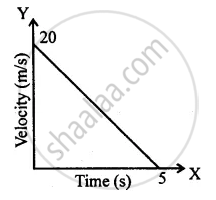• – 4 m/s2

• 4 m/s2

• 10 m/s2

• zero

Unit II Exercise 2 (A) Objective Questions | Q 9

Multiple choice Question. Select the correct option.

The distance covered in the adjoining velocity-time graph is :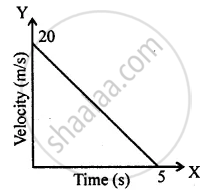• 25 m

• 40 m

• 50 m

• 45 m

Unit II Exercise 2 (A) Objective Questions | Q 10

Multiple choice Question. Select the correct option.

At the maximum height, a body thrown vertically upwards has :

• velocity not zero but acceleration zero.

• acceleration not zero but velocity zero.

• both acceleration and velocity are zero.

• both acceleration and velocity are not zero.

Unit II Exercise 2 (B) Subjective Questions

### Goyal Brothers Prakashan solutions for Class 9 A New Approach to ICSE Physics Part 1 Chapter 2 Motion in One DimensionUnit II Exercise 2 (B) Subjective Questions

Unit II Exercise 2 (B) Subjective Questions | Q 1.1

Draw displacement – time graph for the following situation:

When a body is stationary.

Unit II Exercise 2 (B) Subjective Questions | Q 1.2

Draw displacement – time graph for the following situation:

When a body is moving with uniform velocity.

Unit II Exercise 2 (B) Subjective Questions | Q 1.3

Draw displacement – time graph for the following situation:

When a body is moving with variable velocity.

Unit II Exercise 2 (B) Subjective Questions | Q 2.1

Draw velocity – time graph for the following situation:

When a body, is moving with uniform velocity.

Unit II Exercise 2 (B) Subjective Questions | Q 2.2

Draw velocity – time graph for the following situation:

When a body is moving with variable velocity, but uniform acceleration.

Unit II Exercise 2 (B) Subjective Questions | Q 2.3

Draw velocity – time graph for the following situation:

When a body is moving with variable velocity, but uniform retardation.

Unit II Exercise 2 (B) Subjective Questions | Q 2.4

Draw velocity – time graph for the following situation:

When a body is moving with variable velocity and variable acceleration.

Unit II Exercise 2 (B) Subjective Questions | Q 3.1

How can you find the following?

Velocity from a displacement – time graph.

Unit II Exercise 2 (B) Subjective Questions | Q 3.2

How can you find the following?

Acceleration from velocity – time graph.

Unit II Exercise 2 (B) Subjective Questions | Q 3.3

How can you find the following?

Displacement from velocity – time graph.

Unit II Exercise 2 (B) Subjective Questions | Q 3.4

How can you find the following?

Velocity from acceleration – time graph.

Unit II Exercise 2 (B) Subjective Questions | Q 4.1

Define the term acceleration due to gravity. State its average value.

Unit II Exercise 2 (B) Subjective Questions | Q 4.2

State its value in C.G.S. as well as in S.I. system.

Unit II Exercise 2 (B) Subjective Questions | Q 4.3

When is the acceleration due to gravity positive?

Unit II Exercise 2 (B) Subjective Questions | Q 4.3

When is the acceleration due to gravity negative?

Unit II Exercise 2 (B) Subjective Questions | Q 5.1

Can you suggest the kind of motion of a body from the following distance – time graph?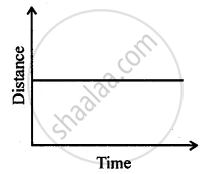Unit II Exercise 2 (B) Subjective Questions | Q 5.2

Can you suggest the kind of motion of a body from the following distance – time graph?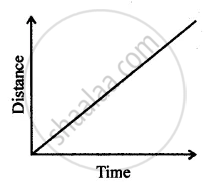Unit II Exercise 2 (B) Subjective Questions | Q 5.3

Can you suggest the kind of motion of a body from the following distance – time graph?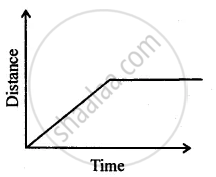Unit II Exercise 2 (B) Subjective Questions | Q 6.1

Can you suggest a real-life example about the motion of a body from the following velocity – time graph?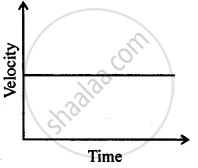Unit II Exercise 2 (B) Subjective Questions | Q 6.2

Can you suggest a real-life example about the motion of a body from the following velocity – time graph?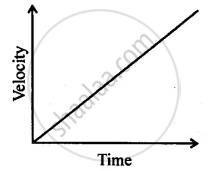Unit II Exercise 2 (B) Subjective Questions | Q 6.3

Can you suggest a real-life example about the motion of a body from the following velocity – time graph?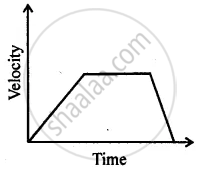Unit II Exercise 2 (B) Subjective Questions | Q 6.4

Can you suggest a real-life example about the motion of a body from the following velocity – time graph?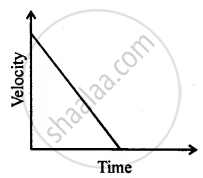Unit II Exercise 2 (B) Subjective Questions | Q 6.5

Can you suggest a real-life example about the motion of a body from the following velocity – time graph?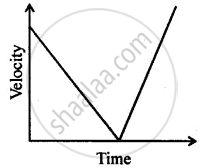Unit II Exercise 2 (B) Subjective Questions | Q 7.1

Diagram shows a velocity – time graph for a car starting from rest. The graph has three sections AB, BC and CD.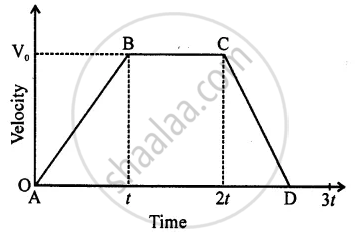From a study of this graph, state how the distance travelled in any section is determined.

Unit II Exercise 2 (B) Subjective Questions | Q 7.2

Diagram shows a velocity – time graph for a car starting from rest. The graph has three sections AB, BC and CD.Compare the distance travelled in section BC with the distance travelled in section AB.

Unit II Exercise 2 (B) Subjective Questions | Q 7.3

Diagram shows a velocity – time graph for a car starting from rest. The graph has three sections AB, BC, and CD.In which section, car has a zero acceleration?

Unit II Exercise 2 (B) Subjective Questions | Q 7.4

Diagram shows a velocity – time graph for a car starting from rest. The graph has three sections AB, BC, and CD.Is the magnitude of acceleration higher or lower than that of retardation? Give a reason.

Unit II Exercise 2 (B) Subjective Questions | Q 8.1

Write down the type of motion of a body along with the A – O – B of the following distance – time graph.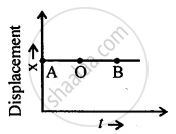Unit II Exercise 2 (B) Subjective Questions | Q 8.2

Write down the type of motion of a body along with the A – O – B of the following distance – time graph.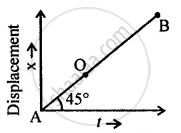Unit II Exercise 2 (B) Subjective Questions | Q 8.3

Write down the type of motion of a body along with the A – O – B of the following distance – time graph.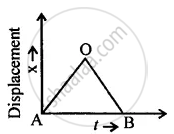Unit II Practice Problems 1

### Goyal Brothers Prakashan solutions for Class 9 A New Approach to ICSE Physics Part 1 Chapter 2 Motion in One DimensionUnit II Practice Problems 1

Unit II Practice Problems 1 | Q 1

From the displacement – time graph shown given below calculate:

1. Average velocity in the first three seconds.
2. Displacement from the initial position at the end of 13 s.
3. Time after which the body is at the initial position,
4. Average velocity after 8 s.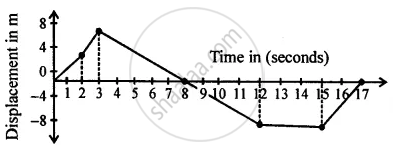Unit II Practice Problems 1 | Q 2

From the displacement – time graph shown given below calculate :

1. Velocity between 0 – 2 s.
2. Velocity between 8 s – 12 s.
3. Average velocity between 5 s – 12 s.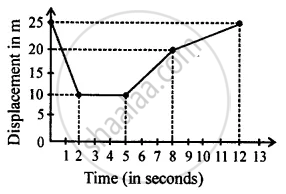Unit II Practice Problems 2

### Goyal Brothers Prakashan solutions for Class 9 A New Approach to ICSE Physics Part 1 Chapter 2 Motion in One DimensionUnit II Practice Problems 2

Unit II Practice Problems 2 | Q 1

A train starting from rest picks up a speed of 20 ms−1 in 200 s. It continues to move at the same rate for the next 500 s and is then brought to rest in another 100 s.

1. Plot a speed-time graph.
2. From graph calculate
(a) uniform rate of acceleration
(b) uniform rate of retardation
(c) total distance covered before stopping
(d) average speed.
Unit II Practice Problems 2 | Q 2

A ball is thrown up vertically and returns back to thrower in 6 s. Assuming there is no air friction, plot a graph between velocity and time. From the graph calculate

1. deceleration
2. acceleration
3. total distance covered by ball
4. average velocity.
Unit II Practice Problems 2 | Q 3

A racing car is moving with a velocity of 50 m/s. On
applying brakes, it is uniformly retarded and comes to rest in 20 seconds. Calculate its acceleration.

Unit II Practice Problems 2 | Q 4

A body falls freely from a certain height. Show graphically the relation between the distance fallen and square of time. How will you determine g from this graph?

Unit II Practice Problems 2 | Q 5

A body at rest is thrown downward from the top of the tower. Draw a distance – time graph of its free fall under gravity during the first 3 seconds. Show your table of values starting t = 0 with an interval of 1 second, (g = 10 ms−2).

Unit II Practice Problems 3

### Goyal Brothers Prakashan solutions for Class 9 A New Approach to ICSE Physics Part 1 Chapter 2 Motion in One DimensionUnit II Practice Problems 3

Unit II Practice Problems 3 | Q 1.1

From the diagram given below, calculate acceleration.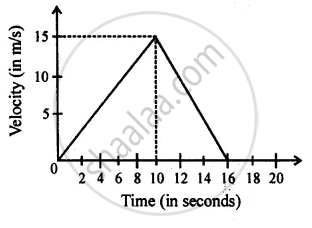Unit II Practice Problems 3 | Q 1.2

From the diagram given below, calculate deceleration.Unit II Practice Problems 3 | Q 1.3

From the diagram given below, calculate distance covered by body.Unit II Practice Problems 3 | Q 2.1

From the velocity – time graph given below, calculate acceleration in the region AB.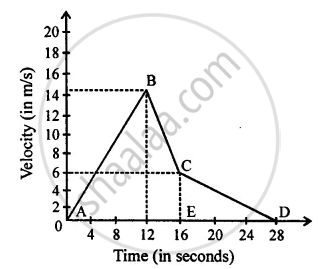Unit II Practice Problems 3 | Q 2.2

From the velocity – time graph given below, calculate deceleration in region BC.Unit II Practice Problems 3 | Q 2.3

From the velocity – time graph given below, calculate Distance covered in the region ABCE.Unit II Practice Problems 3 | Q 2.4

From the velocity – time graph given below, calculate Average velocity in region CED.Unit II Practice Problems 4

### Goyal Brothers Prakashan solutions for Class 9 A New Approach to ICSE Physics Part 1 Chapter 2 Motion in One DimensionUnit II Practice Problems 4

Unit II Practice Problems 4 | Q 1.1

Diagram is given below shows velocity – time graph of car P and Q, starting from the same place and in the same direction. Calculate the Acceleration of car P.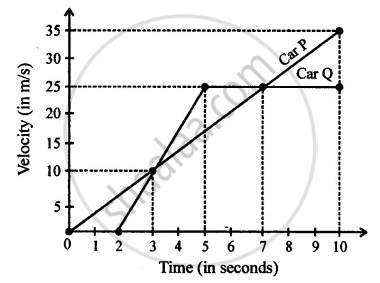Unit II Practice Problems 4 | Q 1.2

Diagram is given below shows velocity – time graph of car P and Q, starting from the same place and in the same direction. Calculate the Acceleration of car Q between 2 s – 5 s.Unit II Practice Problems 4 | Q 1.3

Diagram is given below shows velocity – time graph of car P and Q, starting from the same place and in the same direction. Calculate at what time intervals both cars have the same velocity?Unit II Practice Problems 4 | Q 1.4

Diagram is given below shows velocity – time graph of car P and Q, starting from the same place and in the same direction. Calculate which car is ahead after 10 s and by how much?Unit III Practice Problems 1

### Goyal Brothers Prakashan solutions for Class 9 A New Approach to ICSE Physics Part 1 Chapter 2 Motion in One DimensionUnit III Practice Problems 1

Unit III Practice Problems 1 | Q 1

A motorbike, initially at rest, picks up a velocity of 72 kmh−1 over a distance of 40 m. Calculate

1. acceleration
2. time in which it picks up above velocity.
Unit III Practice Problems 1 | Q 2

A cyclist driving at 5 ms−1, picks a velocity of 10 ms−1, over a distance of 50 m. Calculate

1. acceleration
2. time in which the cyclist picks up above velocity.
Unit III Practice Problems 2

### Goyal Brothers Prakashan solutions for Class 9 A New Approach to ICSE Physics Part 1 Chapter 2 Motion in One DimensionUnit III Practice Problems 2

Unit III Practice Problems 2 | Q 1

An aeroplane lands at 216 kmh−1 and stops after covering a runway of 2 m. Calculate the acceleration and the time, in which it comes to rest.

Unit III Practice Problems 2 | Q 2

A truck running at 90 kmh−1, is brought to rest over a distance of 25 m. Calculate the retardation and time for which brakes are applied.

Unit III Practice Problems 3

### Goyal Brothers Prakashan solutions for Class 9 A New Approach to ICSE Physics Part 1 Chapter 2 Motion in One DimensionUnit III Practice Problems 3

Unit III Practice Problems 3 | Q 1

A racing car, initially at rest, picks up a velocity of 180 kmh−1 in 4.5 s. Calculate

1. acceleration
2. distance covered by the car.
Unit III Practice Problems 3 | Q 2

A motor bike running at 5 ms−1, picks up a velocity of 30 ms−1 in 5s. Calculate

1. acceleration
2. distance covered during acceleration.
Unit III Practice Problems 4

### Goyal Brothers Prakashan solutions for Class 9 A New Approach to ICSE Physics Part 1 Chapter 2 Motion in One DimensionUnit III Practice Problems 4

Unit III Practice Problems 4 | Q 1

A motor bike running at 90 kmh−1 is slowed down to 18 kmh−1 in 2.5 s. Calculate

1. acceleration
2. distance covered during slow down.
Unit III Practice Problems 4 | Q 2

A cyclist driving at 36 kmh−1 stops his motion in 2 s, by the application of brakes. Calculate

1. retardation
2. distance covered during the application of brakes.
Unit III Practice Problems 5

### Goyal Brothers Prakashan solutions for Class 9 A New Approach to ICSE Physics Part 1 Chapter 2 Motion in One DimensionUnit III Practice Problems 5

Unit III Practice Problems 5 | Q 1

A motorbike running at 90 kmh−1, is slowed down to 54 kmh−1 by the application of brakes, over a distance of 40 m. If the brakes are applied with the same force, calculate

1. total time in which bike comes to rest
2. total distance travelled by bike.
Unit III Practice Problems 5 | Q 2

A motor car slows down from 72 kmh−1 to 36 kmh−1 over a distance of 25 m. If the brakes are applied with the same force
calculate

1. total time in which car comes to rest
2. distance travelled by it.
Unit III Practice Problems 6

### Goyal Brothers Prakashan solutions for Class 9 A New Approach to ICSE Physics Part 1 Chapter 2 Motion in One DimensionUnit III Practice Problems 6

Unit III Practice Problems 6 | Q 1

A packet is dropped from a stationary helicopter, hovering at a height ‘h’ from ground level, reaches the ground in 12s. Calculate

1. the value of h
2. final velocity of packet on reaching the ground. (Take g = 9.8 ms−2)
Unit III Practice Problems 6 | Q 2

A boy drops a stone from a cliff, reaches the ground in 8 seconds. Calculate

1. final velocity of stone
2. height of cliff. (Take g = 9.8 ms−2)
Unit III Practice Problems 7

### Goyal Brothers Prakashan solutions for Class 9 A New Approach to ICSE Physics Part 1 Chapter 2 Motion in One DimensionUnit III Practice Problems 7

Unit III Practice Problems 7 | Q 1

A stone thrown vertically upwards takes 3 s to attain maximum height. Calculate

1. initial velocity of the stone
2. maximum height attained by the stone. (Take g = 9.8 ms−2)
Unit III Practice Problems 7 | Q 2

A stone thrown vertically upwards takes 4 s to return to the thrower. Calculate

1. initial velocity of the stone
2. maximum height attained by stone. (Take g = 10 ms−2)
Unit III Practice Problems 8

### Goyal Brothers Prakashan solutions for Class 9 A New Approach to ICSE Physics Part 1 Chapter 2 Motion in One DimensionUnit III Practice Problems 8

Unit III Practice Problems 8 | Q 1

A spaceship is moving in space with a velocity of 50 kms−1. Its engine fires for 10 s, such that its velocity increases to 60 kms−1. Calculate the total distance travelled by a spaceship in 1/2 minute, from the time of firing its engine

Unit III Practice Problems 8 | Q 2

A spaceship is moving in space with a velocity of 60 kms−1. It fires its retro engines for 20 seconds and velocity is reduced to 55 kms−1. Calculate the distance travelled by a spaceship in 40 s, from the time of firing of the retro- rockets.

## Chapter 2: Motion in One Dimension

Unit I Exercise 1ExerciseUnit I Practice Problems 1Unit I Practice Problems 2Unit II Exercise 2 (A) Objective QuestionsUnit II Exercise 2 (B) Subjective QuestionsUnit II Practice Problems 1Unit II Practice Problems 2Unit II Practice Problems 3Unit II Practice Problems 4Unit III Practice Problems 1Unit III Practice Problems 2Unit III Practice Problems 3Unit III Practice Problems 4Unit III Practice Problems 5Unit III Practice Problems 6Unit III Practice Problems 7Unit III Practice Problems 8## Goyal Brothers Prakashan solutions for Class 9 A New Approach to ICSE Physics Part 1 chapter 2 - Motion in One Dimension

Goyal Brothers Prakashan solutions for Class 9 A New Approach to ICSE Physics Part 1 chapter 2 (Motion in One Dimension) include all questions with solution and detail explanation. This will clear students doubts about any question and improve application skills while preparing for board exams. The detailed, step-by-step solutions will help you understand the concepts better and clear your confusions, if any. Shaalaa.com has the CISCE Class 9 A New Approach to ICSE Physics Part 1 solutions in a manner that help students grasp basic concepts better and faster.

Further, we at Shaalaa.com provide such solutions so that students can prepare for written exams. Goyal Brothers Prakashan textbook solutions can be a core help for self-study and acts as a perfect self-help guidance for students.

Concepts covered in Class 9 A New Approach to ICSE Physics Part 1 chapter 2 Motion in One Dimension are Scalar and Vector Quantities, Graphical Representation of Motion: Speed - Time Graphs, Measuring the Rate of Motion - Speed with Direction, Rate of Change of Velocity, Instantaneous Velocity and Speed, Acceleration and Retardation, Distance and Displacement.

Using Goyal Brothers Prakashan Class 9 solutions Motion in One Dimension exercise by students are an easy way to prepare for the exams, as they involve solutions arranged chapter-wise also page wise. The questions involved in Goyal Brothers Prakashan Solutions are important questions that can be asked in the final exam. Maximum students of CISCE Class 9 prefer Goyal Brothers Prakashan Textbook Solutions to score more in exam.

Get the free view of chapter 2 Motion in One Dimension Class 9 extra questions for Class 9 A New Approach to ICSE Physics Part 1 and can use Shaalaa.com to keep it handy for your exam preparation

Advertisement Remove all ads
Share
Notifications

View all notifications

Forgot password?